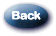# What are binary, octal, and hexadecimal notations?

What are binary, octal, and hexadecimal notations?

Binary Notation

All data in modern computers is stored as series of bits. A bit can take on one of two values. The two values are generally represented as the numbers 0 and 1. The most basic form of representing computer data, then, is to represent a piece of data as a string of 1’s and 0’s, one for each bit. What you end up with is a binary, or base-2 number; this is binary notation. For example, the number 42 would be represented in binary as

101010

Interpreting Binary Notation

As with normal decimal (base-10) notation each digit moving from right to left represents an increasing order of magnitude (or power of ten). With decimal notation each succeeding digit’s contribution is ten times greater than the previous digit. Increasing the first digit by one increases the number represented by one, increasing the second digit by one increases the number by ten, the third digit increases the number by 100, and so on. The number 111 is one less than 112, ten less than 121, and one hundred less than the number 211.

The concept is the same with binary notation except that each digit is a power of two greater than the preceding digit rather than a power of ten. Instead of 1’s, 10’s, 100’s, and 1000’s digits, binary numbers have 1’s, 2’s, 4’s, and 8’s. Thus, the number two in binary would be represented as a 0 in the ones place and a 1 in the twos place, i.e., 10. Three would be 11, a one in the ones place and a 1 in the twos place. No numeral greater than 1 is ever used in binary notation.

Since binary notation can be cumbersome, two more compact notations are often used, octal and hexadecimal. Octal notation represents data as a base-8 number. Each digit in an octal number represents three bits. Similarly, hexadecimal notation uses base-16 numbers, representing four bits with each digit. Octal numbers use only the digits 0-7, while hexadecimal numbers use all ten base-10 digits (0-9) and the letters a-f (represent the numbers 10-15). The number 42 is written in octal as

52

2a

It can sometimes be difficult to tell whether data is being represented as octal, or hexadecimal (especially if a hexadecimal number doesn’t use one of the digits 8-f), so one convention that is often used to distinguish these is to put `0x` in front of hexadecimal numbers. Thus, you will often see

0x2a

as another less ambiguous way of representing the number 42 in hexadecimal. An example of this usage can be seen here: Character set comparison chart.

Note: The term binary when used in phrases such as “binary” or “binary attachment” has a related but slightly different meaning than the one discussed here.

What is a binary file?

A binary file is one in which the eighth bit of each byte is used for data. Computers and programs can read binary files, but people cannot. Executable files, compiled programs, WordPerfect documents, SAS and SPSS system files, and spreadsheets are all examples of binary files.

Files that contain machine-specific codes (i.e., processor-specific microcode) are binary files. However, not all binary files contain processor-specific codes. Some binary files contain text or data in a non-ASCII format that is unrelated to the microcode used by the processor. For example, most graphics files, all compressed files, and many other file types use all eight bits per byte, so are termed “binary”.

What is a bit?

A bit is a binary digit, the smallest increment of data on a machine. A bit can hold only one of two values: 0 or 1.

Because bits are so small, you rarely work with information one bit at a time. Bits are usually assembled into a group of 8 to form a byte. A byte contains enough information to store a character, like “h”.

What is a byte?

Byte is an abbreviation for “binary term”. A single byte is composed of 8 consecutive bits capable of storing a single character.